# 使用Python中的卷积神经网络进行恶意软件检测1import numpy from keras.datasets import mnist from keras.models import Sequential from keras.layers import Dense from keras.layers import Dropout from keras.layers import Flatten from keras.layers.convolutional import Conv2D from keras.layers.convolutional import MaxPooling2D from keras.utils import np_utils from keras import backend backend.set_image_dim_ordering('th') model = Sequential() model.add(Conv2D(32, (5, 5), input_shape = (1, 28, 28), )) model.add(MaxPooling2D(pool_size = (2, 2))) model.add(Dropout(0.2)) model.add(Flatten()) model.add(Dense(128, )) model.add(Dense(num_classes, )) model.compile(, , metrics = ['accuracy'])import numpy from keras.datasets import mnist from keras.models import Sequential from keras.layers import Dense from keras.layers import Dropout from keras.layers import Flatten from keras.layers.convolutional import Conv2D from keras.layers.convolutional import MaxPooling2D from keras.utils import np_utils from keras import backend backend.set_image_dim_ordering('th') model = Sequential() model.add(Conv2D(32, (5, 5), input_shape = (1, 28, 28), )) model.add(MaxPooling2D(pool_size = (2, 2))) model.add(Dropout(0.2)) model.add(Flatten()) model.add(Dense(128, )) model.add(Dense(num_classes, )) model.compile(, , metrics = ['accuracy'])

RNN对序列的每个元素执行相同的任务，输出取决于先前的计算。它广泛应用于语言建模和文本生成（机器翻译、语音识别和许多其他应用程序）。

Hopfield网络

Hopfield网络由John Hopfield于1982年开发。这个网络的主要目标是自动关联和优化，分为两类：离散和连续。

Boltzmann机器网络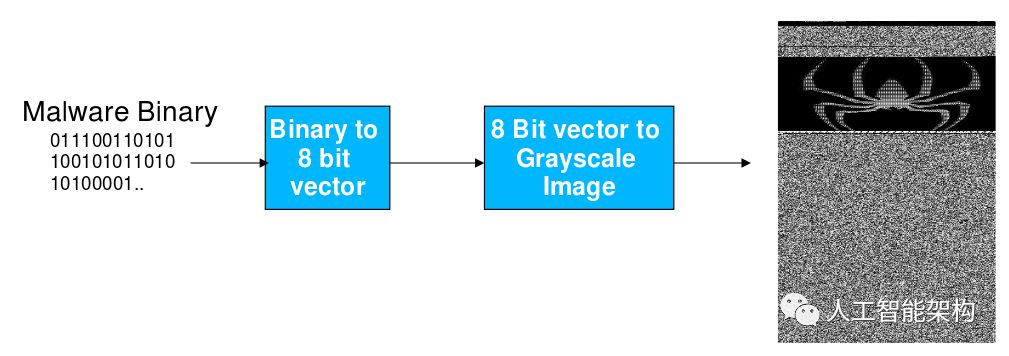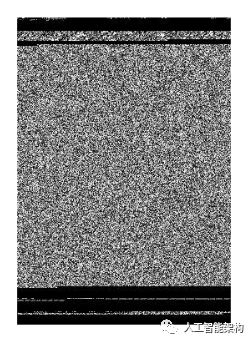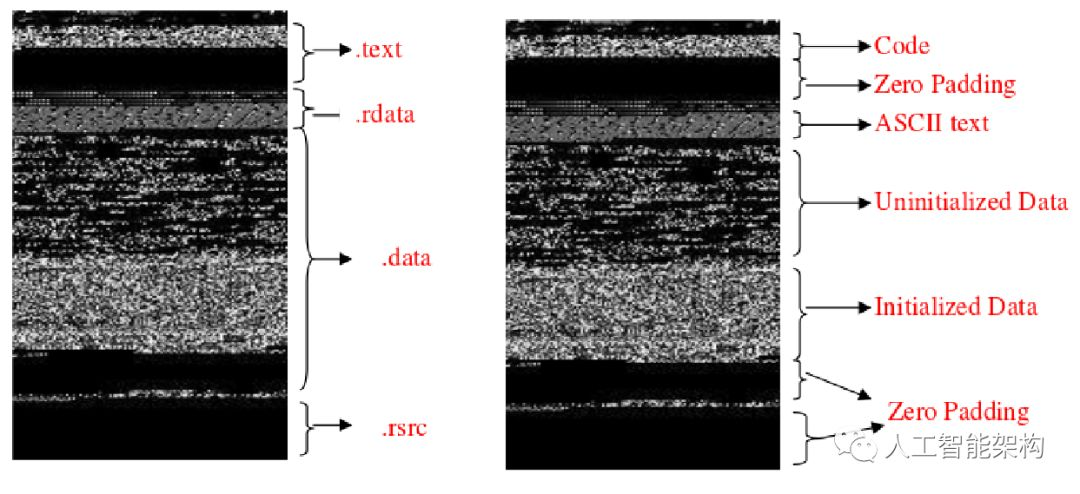Allaple.L

Allaple.A

Yuner.A

Lolyda.AA 1

Lolyda.AA 2

Lolyda.AA 3

C2Lop.P

C2Lop.gen!G

Instant access

Swizzor.gen!I

Swizzor.gen!E

VB.AT

Fakerean

Alueron.gen!J

Malex.gen!J

Lolyda.AT

Wintrim.BX

Dialplatform.B

Dontovo.A

Agent.FYI

Autorun.K

Rbot!gen

Skintrim.N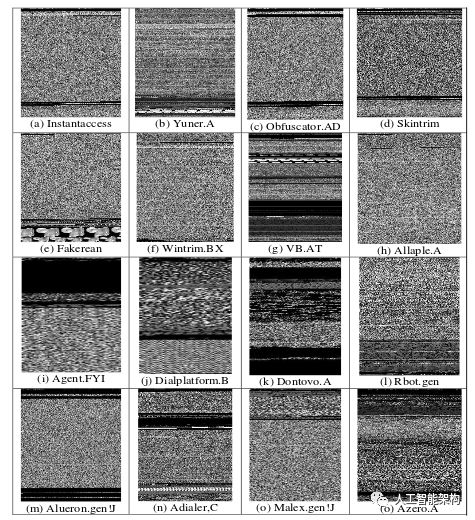1import os 2import scipy 3import array 4filename = '<Malware_File_Name_Here>'; 5f = open(filename,'rb'); 6ln = os.path.getsize(filename); 7width = 256; 8rem = ln%width; 9a = array.array("B");10a.fromfile(f,ln-rem);11f.close();12g = numpy.reshape(a,(len(a)/width,width));13g = numpy.uint8(g);14scipy.misc.imsave('<Malware_File_Name_Here>.png',g);import os 2import scipy 3import array 4filename = '<Malware_File_Name_Here>'; 5f = open(filename,'rb'); 6ln = os.path.getsize(filename); 7width = 256; 8rem = ln%width; 9a = array.array("B");10a.fromfile(f,ln-rem);11f.close();12g = numpy.reshape(a,(len(a)/width,width));13g = numpy.uint8(g);14scipy.misc.imsave('<Malware_File_Name_Here>.png',g);

1# pip install pyleargist=.0.1# pip install pyleargist=.0.1

1import Image2Import leargist3image = Image.open('<Image_Name_Here>.png');4New_im = image.resize((64,64));5des = leargist.color_gist(New_im);6Feature_Vector = des[0:320];import Image2Import leargist3image = Image.open('<Image_Name_Here>.png');4New_im = image.resize((64,64));5des = leargist.color_gist(New_im);6Feature_Vector = des[0:320];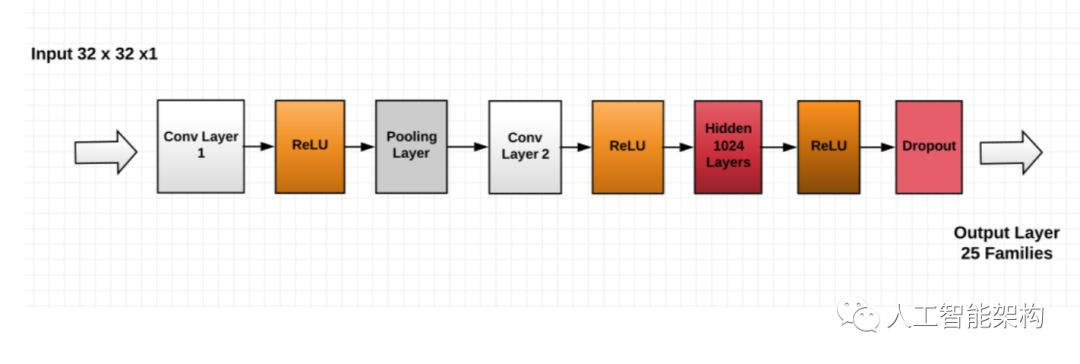1import keras 2from keras.models 3import Sequential, Input, Model 4from keras.layers 5import Dense, Dropout, Flatten 6from keras.layers 7import Conv2D, MaxPooling2D 8from keras.layers.normalization 9import BatchNormalization10from keras.layers.advanced_activations11import LeakyReLUimport keras 2from keras.models 3import Sequential, Input, Model 4from keras.layers 5import Dense, Dropout, Flatten 6from keras.layers 7import Conv2D, MaxPooling2D 8from keras.layers.normalization 9import BatchNormalization10from keras.layers.advanced_activations11import LeakyReLU

1train_X = train_X.reshape(-1, 32,32, 1)2test_X = test_X.reshape(-1, 32,32, 1)-1, 32,32, 1)2test_X = test_X.reshape(-1, 32,32, 1)

1batch_size = 642epochs = 203num_classes = 25642epochs = 203num_classes = 25

 1Malware_Model = Sequential() 2Malware_Model.add(Conv2D(32, kernel_size=(3,3),,input_shape=(32,32,1),)) 3Malware_Model.add(LeakyReLU(.1)) 4Malware_model.add(MaxPooling2D(pool_size=(2, 2),)) 5Malware_Model.add(Conv2D(64, (3, 3), ,)) 6Malware_Model.add(LeakyReLU(.1)) 7Malware_Model.add(Dense(1024, )) 8Malware_Model.add(LeakyReLU(.1)) 9Malware_Model.add(Dropout(0.4))10Malware_Model.add(Dense(num_classes, )) 2Malware_Model.add(Conv2D(32, kernel_size=(3,3),,input_shape=(32,32,1),)) 3Malware_Model.add(LeakyReLU(.1)) 4Malware_model.add(MaxPooling2D(pool_size=(2, 2),)) 5Malware_Model.add(Conv2D(64, (3, 3), ,)) 6Malware_Model.add(LeakyReLU(.1)) 7Malware_Model.add(Dense(1024, )) 8Malware_Model.add(LeakyReLU(.1)) 9Malware_Model.add(Dropout(0.4))10Malware_Model.add(Dense(num_classes, ))

1Malware_Model.compile(.losses.categorical_crossentropy, .optimizers.Adam(),metrics=['accuracy'])'accuracy'])

1Malware_Model.fit(train_X, train_label, ,,,validation_data=(valid_X, valid_label))

1Malware_Model.evaluate(test_X, test_Y_one_hot, )2print('The accuracy of the Test is:', test_eval)2print('The accuracy of the Test is:', test_eval)10-2198
07-013119
04-051213
04-20
01-05847
12-30
06-012161
07-311563
12-04387
11-185856
06-22
04-01
05-21386

### “相关推荐”对你有帮助么？

•非常没帮助
•没帮助
•一般
•有帮助
•非常有帮助被折叠的  条评论 为什么被折叠?到【灌水乐园】发言点击重新获取扫码支付1.余额是钱包充值的虚拟货币，按照1:1的比例进行支付金额的抵扣。
2.余额无法直接购买下载，可以购买VIP、付费专栏及课程。余额充值